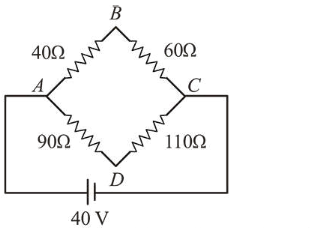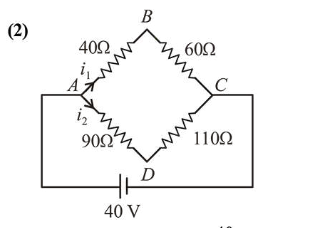# Solve thisQuestion:Four resistances $40 \Omega, 60 \Omega, 90 \Omega$ and $110 \Omega$ make the arms of a quadrilateral $A B C D$. Across $A C$ is a battery of emf $40 \mathrm{~V}$ and internal resistance negligible. The potential difference across $B D$ in $\mathrm{V}$ is ___________

Solution:Current through $A B, i_{1}=\frac{40}{40+60}=0.4$

Current through $A D, i_{2}=\frac{40}{90+110}=\frac{1}{5}$

$V_{B}+i_{1}(40)-i_{2}(90)=V_{D}$
$\Rightarrow V_{B}-V_{D}=\frac{1}{5}(90)-\frac{4}{10}(40)$
$\Rightarrow V_{B}-V_{D}=18-16=2 \mathrm{~V}$